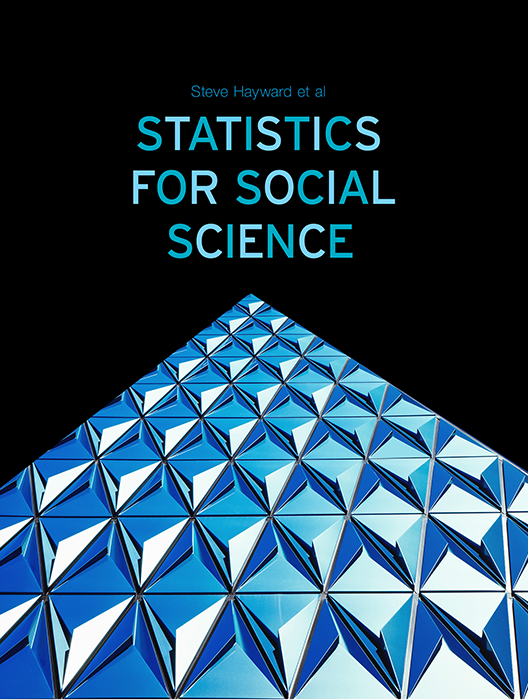# Statistics for Social Science

Statistics for Social Science takes a fresh approach to the introductory class. With learning check questions, embedded videos and interactive simulations, students engage in active learning as they read. An emphasis on real-world and academic applications help ground the concepts presented. Designed for students taking an introductory statistics course in psychology, sociology or any other social science discipline.

## What is a Top Hat Textbook?

Top Hat has reimagined the textbook – one that is designed to improve student readership through interactivity, is updated by a community of collaborating professors with the newest information, and accessed online from anywhere, at anytime.

• Top Hat Textbooks are built full of embedded videos, interactive timelines, charts, graphs, and video lessons from the authors themselves
• High-quality and affordable, at a significant fraction in cost vs traditional publisher textbooks

## Key features in this textbookOur Statistics for Social Science textbook allows students to manipulate data, visualize the effects discussed, and explore Lightboard videos that feature instructor explanations to reinforce concepts and calculations.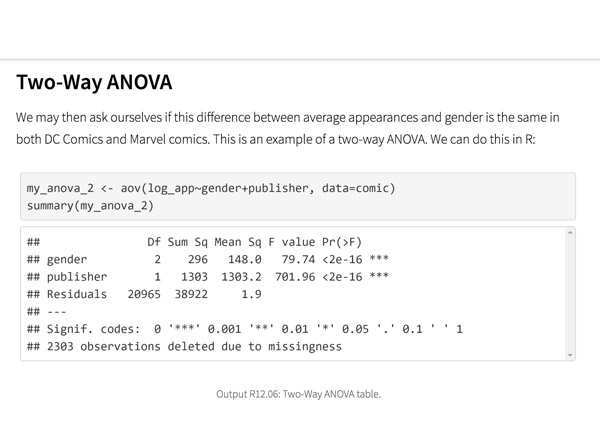Top Hat’s interactive offering includes a complementary module on using R software for data management, graphics, and conducting statistical analyses with examples and practice questions.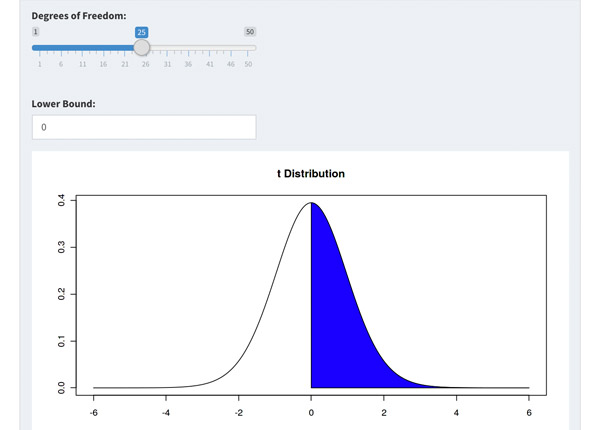Built-in assessment questions embedded throughout chapters so students can read a little, do a little, and test themselves to see what they know!

## Comparison of Social Sciences Textbooks

Consider adding Top Hat’s Statistics for Social Sciences textbook to your upcoming course. We’ve put together a textbook comparison to make it easy for you in your upcoming evaluation.

### Top Hat

Steve Hayward et al., Statistics for Social Sciences, Only one edition needed

### Pearson

Agresti, Statistical Methods for the Social Sciences, 5th Edition

### Cengage

Gravetter et al., Essentials of Statistics for The Behavioral Sciences, 9th Edition

### Sage

Gregory Privitera, Essentials Statistics for the Behavioral Sciences, 2nd Edition

### Pricing

Average price of textbook across most common format

#### $200.83 Hardcover print text only ####$239.95

Hardcover print text only

#### $92 Hardcover print text only ### Always up-to-date content, constantly revised by community of professors Content meets standard for Introduction to Anatomy & Physiology course, and is updated with the latest content ### In-Book Interactivity Includes embedded multi-media files and integrated software to enhance visual presentation of concepts directly in textbook Only available with supplementary resources at additional cost Only available with supplementary resources at additional cost Only available with supplementary resources at additional cost ### Customizable Ability to revise, adjust and adapt content to meet needs of course and instructor ### All-in-one Platform Access to additional questions, test banks, and slides available within one platform ## Pricing Average price of textbook across most common format ### Top Hat Steve Hayward et al., Statistics for Social Sciences, Only one edition needed #### Up to40-60%more affordable Lifetime access on any device ### Pearson Agresti, Statistical Methods for the Social Sciences, 5th Edition ####$200.83

Hardcover print text only

### Pearson

Gravetter et al., Essentials of Statistics for The Behavioral Sciences, 9th Edition

#### $239.95 Hardcover print text only ### Sage McConnell, Brue, Flynn, Principles of Microeconomics, 7th Edition ####$92

Hardcover print text only

## Always up-to-date content, constantly revised by community of professors

Constantly revised and updated by a community of professors with the latest content

### Top Hat

Steve Hayward et al., Statistics for Social Sciences, Only one edition needed

### Pearson

Agresti, Statistical Methods for the Social Sciences, 5th Edition

### Pearson

Gravetter et al., Essentials of Statistics for The Behavioral Sciences, 9th Edition

### Sage

Gregory Privitera, Essentials Statistics for the Behavioral Sciences, 2nd Edition

## In-book Interactivity

Includes embedded multi-media files and integrated software to enhance visual presentation of concepts directly in textbook

### Top Hat

Steve Hayward et al., Statistics for Social Sciences, Only one edition needed

### Pearson

Agresti, Statistical Methods for the Social Sciences, 5th Edition

### Pearson

Gravetter et al., Essentials of Statistics for The Behavioral Sciences, 9th Edition

### Sage

Gregory Privitera, Essentials Statistics for the Behavioral Sciences, 2nd Edition

## Customizable

Ability to revise, adjust and adapt content to meet needs of course and instructor

### Top Hat

Steve Hayward et al., Statistics for Social Sciences, Only one edition needed

### Pearson

Agresti, Statistical Methods for the Social Sciences, 5th Edition

### Pearson

Gravetter et al., Essentials of Statistics for The Behavioral Sciences, 9th Edition

### Sage

Gregory Privitera, Essentials Statistics for the Behavioral Sciences, 2nd Edition

## All-in-one Platform

### Top Hat

Steve Hayward et al., Statistics for Social Sciences, Only one edition needed

### Pearson

Agresti, Statistical Methods for the Social Sciences, 5th Edition

### Pearson

Gravetter et al., Essentials of Statistics for The Behavioral Sciences, 9th Edition

### Sage

Gregory Privitera, Essentials Statistics for the Behavioral Sciences, 2nd Edition

A lifelong learner, Steve focused on statistics and research methodology during his graduate training at the University of New Mexico. He later founded and served as CEO of the Center for Performance Technology, providing instructional design and training development support to larger client organizations throughout the United States. Steve is presently a lead faculty member for statistics at Rio Salado College in Tempe, Arizona.

## Explore this textbook

Read the fully unlocked textbook below, and if you’re interested in learning more, get in touch to see how you can use this textbook in your course today.

# Discrete Probability DistributionsAlbert Harris and his Coconut Shy at the Cambridge Midsummer Fair 2005. Are carnival game outcomes "random"? ​

## Chapter Objectives

After completing this chapter, you will be able to:

• Identify what makes a variable “random.”
• Describe the difference between discrete and continuous variables.
• Describe a probability distribution and explain what information it conveys about a variable.
• Explain the Binomial distribution and provide examples.
• Compute probabilities associated with the binomial distribution.
• Explain the difference between the binomial and multinomial distribution.

## What is a Random Variable?

In probability and statistics, many scenarios that exhibit the same characteristics can be considered, so probabilities can be calculated utilizing a formula that is derived once and then utilized for all scenarios with the same characteristics. Variables are left as “to be determined” and represented by letters but can be used in computations in most of the same ways that numbers can. Then, when we have a value, we can plug it in and get a solution. Using equations with variables—formulas—allows us to state a general relationship to which specific values can be applied.

The use of variables as placeholders in formulas is particularly important in probability and statistics, as we most often want to use multiple instances of a given variable or variables. For example, if we want to determine the extent to which a person’s height and shoe size are related to one another, we need lots of examples of those two paired values obtained from many individuals. Variables can be used as simple placeholders until a value is given, but more importantly for our purposes, variables allow us to consider multiple instances, with values of the variable assigned according to the scenario at hand.

Example

Below is an example of a formula that summarizes the relationship between two measures. In this case, the formula can be used to convert from degrees Fahrenheit to degrees Centigrade. In this formula, f can be replaced by any Fahrenheit value, and carrying out the calculation yields a result expressed in degrees Centigrade.

If you plug in 32º for the f, or Fahrenheit variable, we can readily compute that the Centigrade counterpart is 0°. The formula enables us to take any Fahrenheit value we have at any time and determine the Centigrade equivalent.

Variables, like numbers, have algebraic qualities: For instance, we can do some very simple algebraic operations, and solve for f (get f on one side of the equation by itself) so that we can now start with a Centigrade measurement and find the Fahrenheit equivalent.

​A random variable is a numerical value representing the outcome of a probability experiment. The classical definition is that a random variable is a rule that assigns a numerical value to every outcome in the sample space. It is a random variable if the value it takes on is determined entirely by chance factors, or probabilistic factors. If you throw 1,000 pennies onto the floor and count up the number of heads, the variable “number of heads” is a random variable with anything between zero heads and 1000 heads being possible values. Some people mistakenly think that a variable is random if each possible outcome has an equal likelihood. This is not the case. With our pennies example, the probability of zero or 1000 heads is vanishingly unlikely, while values closer to the middle—around 500 heads—are the most likely values. We’ll discuss why this is the case shortly.

5.01

Which of the following is not an example of a random variable?

A

The number that comes up in a single toss of a die

B

Hair color of a person selected at random

C

The final number in a lottery draw

D

The sum total of the numbers in a lottery draw

Speaking of the lottery, have you ever wondered what a statistician's opinion of it is? Take a look below:

5.02

Variables act as $\_\_\_\_\_\_\_$ for numerical values in formulas.

5.03

What is the most likely value of the random variable “number of heads” resulting from ten tosses of a fair coin?

## Discrete vs. Continuous Random Variables

### Discrete Random Variables

In games of chance, most variables are discrete in that they can take on only a finite number of fixed values, with no “inbetweens.” If you draw a card from a stack of cards that includes the ace through 10 of hearts, there are ten and only ten possible outcomes. If you reach your hand into a bag of pennies and pull out a handful, the number of pennies you have will be a whole number and cannot be anything in between.

The key to identifying a discrete random variable is that the outcomes can be counted.

### Continuous Random Variables

In nature, most of the variables we encounter are continuous rather than discrete. Consider, for example, the variable height. It is continuous in that it can take on any possible value within a given interval. The variable is not restricted to specific values; therefore, the possible values of the variable cannot be counted.

A continuous random variable has an uncountable number of possible outcomes. The outcomes can be measured.

Examples

5.04

Without regards to limitations of the measurement device, which of the following is a discrete random variable?

A

Speed of an automobile

B

Wind velocity

C

Number of finishers in a race

D

Elapsed time to run a quarter mile

5.05

The outcomes of a discrete random variable can be $\_\_\_\_\_\_.$

Do people use probability distributions in real life? Can you imagine if we could use them to predict where crimes will occur and prevent them before they even happen?

## Discrete Probability Distributions

A probability distribution for a discrete random variable is simply a list of every possible value that the variable can take on and the accompanying probability for each of those possible outcomes. One of the most central ideas of probability is that some outcomes are more likely than others. This can best be seen in either table or graph format.

A discrete probability distribution includes each value the variable can take on (as above), along with the probability of each of those values. It must satisfy two conditions:

### How to Construct a Discrete Probability Distribution

A discrete probability distribution can be summarized in a frequency distribution table or chart like those you’ve already seen. The table below summarizes the steps to follow to construct a probability distribution for a random variable with possible outcomes expressed as x1, x2 . . . xn.

### Examples of Discrete Probability Distributions

Example 1: Dandelions in Parks

Suppose that in order to determine the extent of a dandelion problem for a given town’s parks, 500 grass patches, each one square yard, were randomly sampled. In each grass patch the number of dandelions was carefully counted. Then the number of dandelions in a given patch is the variable X. Sometimes no dandelions were present, sometimes as many as 14. All of the patches sampled were sorted by how many dandelions they contained and the proportion (or probability) of a patch having X dandelions was tabulated and graphed as below. The resulting frequency table summarizing the outcomes is shown below, followed by a chart displaying the probabilities.

Example 2: Studying Habits

A psychology instructor surveyed students’ studying habits to find how much time students spent studying the day before a major exam. Here are the raw data, expressed as hours studying: 7, 4, 1, 7, 5, 9, 8, 3, 6, 6, 3, 8, 4, 8, 6, 5, 7, 3, 2, 6.

### How to Find the Mean and Standard Deviation of a Probability Distribution

The mean of a probability distribution can be found using the formula μ = ∑ x P(x). The procedure for finding the variance is very similar to the procedure you learned in Lesson Two and uses the formula σ2 = ∑ P(x) (x – μ)2. The standard deviation is the square root of the variance, just as before.

Example

Step 1: Using the data from the previous example, the mean can be found by summing the products of x and P(x).

Step 2: The mean of the probability distribution is μ = ∑ x P(x) = 5.4

Step 3: Once you have the mean, you can calculate the variance by summing the products of P(x) and (x – μ)2.

Step 4: The variance of the probability distribution is σ2 = ∑ P(x) (x – μ)2 = 4.74

Step 5: The standard deviation is σ = √4.74 = 2.18

### Shortcut Formula for the Variance

There is a shortcut formula for the variance that will give you the same result. This is the formula used by most calculators.

### Expected Value

The expected value of a probability distribution is the value you would expect to get on any given trial of the experiment. The values will vary on a trial-by-trial basis, but over the long run will be very close to the mean. The expected value is given as

Knowing how to figure the expected value of a probability distribution can be especially useful in determining the outcome to expect from a distribution with multiple events.

Note that while an individual probability can never be less than zero, an expected value of a random variable can be negative (since a random variable can be either positive or negative).

Example

Let’s say you buy a $10 raffle ticket for a new car that is valued at$15,000. Two thousand tickets are sold. What is the expected value of your gain or loss?

### Solution

Step 1: Figure the probability of each outcome, win or lose

Step 2: Multiply x and P(x).

Step 3: Sum the products to get the mean, which represents the expected outcome. In this case, you expect to lose $2.50 for each ticket purchased! 5.06 Identify the correct statement. A $P$($x$) ≤ 1 B $P$($x$) = 1 C $P$($x$) ≥ 1 D $P$($x$) ≠ 1 5.07 The expected value of a probability distribution is equal to the $\_\_\_\_\_\_.$ 5.08 Calculate the probability of getting less than 2 heads in 4 tosses of a fair coin. ## ​About Factorials! A variable followed by “!” is called a factorial. This is a fairly simple computational element; many calculators and all spreadsheets have a formula for it. Here’s how it works. You begin with the stated value (variable) and multiply it by 1 less than itself, then 2 less than itself, and so forth until you reach the value 1, at which point you stop. ### Examples of Factorial Calculation Calculating factorials for small numbers is easy enough, but as the stated value increases, the calculations rapidly become more complex. For example, 3! = 3 × 2 × 1 = 6 5! = 5 × 4 × 3 × 2 × 1 = 120 8! = 8 × 7 × 6 × 5 × 4 × 3 × 2 × 1 = 40,320 You can see from these examples that the resulting calculations soon become very cumbersome, resulting in some really large numbers. For that reason, it is generally best to use a technological tool when calculating factorials. There are also online calculators available on the web; one such is available here. ### Zero Factorial = 0! = 1 For the case of zero successes, you will need a value for zero factorial, 0!. Zero factorial is defined as 1, so that part is easy enough, if not very intuitive. If you’d like to explore the rationale for that, there is a link to an online discussion here. ## Permutations and Combinations We can take this a step further by examining the procedures for calculating permutations and combinations. ### Permutations A permutation is a set of k items chosen from a set of n items without replacement and where order does matter. It is a specific arrangement of all or part of the n items. With permutations, the list A, B, C, is not the same as B, A, C. The possible number of permutations of a given set can be calculated by the formula where n equals the number of items to be selected from and k equals the number of items to be chosen and ordered. We can start by considering the possible number of permutations of an entire set. In that case, P(n,n) = n! In other words, if we were to get all possible permutations of an entire set of n items, the total of the permutation would be equal to n! The rationale for this is that there are n ways to choose the first item, n-1 ways to choose the second, n-2 ways to choose the third, and so forth down to the last item. The general rule is that: The number of permutations of n items taken n at a time is n! Example Let n = 4. From a group of 4 items, there are 4 ways to choose the first item, then 3 ways to choose the second, 2 ways to choose the third and only one way to choose the fourth. This works out to 4 × 3 × 2 × 1 = 24, which is equal to 4! From that, then, we can see that P(n,n) = P(4,4) = 4!. We can confirm this by substituting in the formula: Next, let’s consider the possible number of permutations of less than an entire set. The general rule in this case is that: The number of permutations of n items taken k at a time is: Example Let n = 4 and k = 2. From a group of 4 items there are 4 ways to choose the first item and 3 ways to choose the second. This works out to 4 × 3 = 12 possible permutations of 4 items taken 2 at a time. We can confirm this by again substituting in the formula: ### Combinations A combination is a set of k items chosen from a set of n items without replacement and where the order does not matter. With combinations, the group "A, B, C" is the same as the group "B, A, C". The possible number of combinations of a given set can be calculated by the formula where n equals the number of items to be selected from and k equals the number of items to be chosen without regards to order. Since order is not important in determining combinations, the number of possible combinations will be less than the number of possible permutations. The combinations are included among the permutations and are in effect a subset of the permutations. Example Following the formulas above, we can determine the permutations and combinations possible from the same set of items, a, b, c, d. They tell us that To confirm the calculations, we can construct a table:Figure 5.10: Combinations vs. permutations From this table, we can readily see that from a set of four items taken three at a time, we can construct 24 distinct permutations but only four distinct combinations! 5.09 Which of the following scenarios represent permutations? A Creating a password using 6 letters B Choosing 3 ice cream flavors from a list of 12 C Assigning players to a batting order on a baseball team D Selecting 8 volunteers for a task from a pool of 20 5.10 A combination is sensitive to order. A True B False 5.11 Calculate C(n,k) where n=9 and k=3. ## Binomial Experiment A binomial experiment must satisfy four conditions • It has a fixed number of trials • Each trial is independent of the other trials • There are only two possible outcomes • The probability of each outcome is constant from trial to trial These outcomes are often referred to as success and failure, respectively. In standard statistical notation, the probability of success is represented as p and the probability of failure is represented as q, and the condition p + q = 1 must be met. I.e., p and q account for all possible outcomes. Additional necessary terms are n, the number of trials, and k, representing the binomial random variable given as the number of successes in n trials. The notation used for a binomial experiment is summarized in the table below:Figure 5.11: Binomial experiment notation ### Binomial Distribution The Binomial Distribution is a probability distribution summarizing the outcomes of a binomial experiment. It must satisfy these conditions: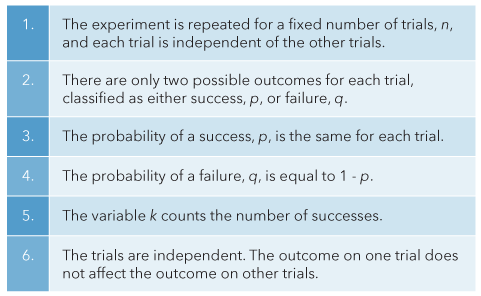Figure 5.12: Conditions for a binomial distribution ### Binary Data A binomial distribution is made up of binary data, like zero or one, yes or no, pass or fail, accept or reject, or success or failure. When working with binary data, you only know whether an event happened and not the magnitude of the event. ### The Binomial Coefficient The binomial coefficient is represented as and is stated as “n choose k.” It represents the number of possible ways to choose k successes from n observations. The formula for the calculation is: You will notice that is exactly the same formula as was given for combinations. In other words, the binomial coefficient is really just a way of determining combinations (ways of choosing k successes from n trials), and the formulas are equivalent, so Here is a procedure to follow in carrying out the calculations: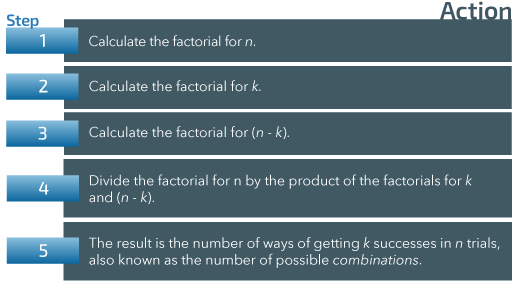Figure 5.13: Steps for calculating the binomial coefficient​ 5.12 The binomial distribution is a type of $\_\_\_\_\_\_\_$ distribution. A Probability B Continuous C Non-specific D Random 5.13 In generating a binomial probability distribution, each trial must be $\_\_\_\_\_\_$ of any other trial. 5.14 Each trial in a binomial probability distribution has how many possible outcomes? ## Probabilities of Outcomes in Binomial Trials We can calculate the probability of observing a specific number of successes when the process is repeated a specific number of times and each outcome can be identified as either a success or failure. The calculation involves only three basic elements: the binomial coefficient (which we’ve seen demonstrated earlier), the probability of success, p, and the probability of failure, q. They are combined as: Example Let’s say you want to determine the probability of heads coming up exactly two times in four tosses of a fair coin. Since the coin is fair, meaning unbiased, we know there is an equal chance of either heads or tails coming up on any toss. Given that, the probability of heads coming up on any given toss, represented by p, is .5. p = .5 q = 1 – p = .5 The number of trials, n, is equal to 4 and the number of successes, k, is equal to 2. To start the process, you first need to calculate the combinations—the number of ways of getting 2 successes in 4 trials—represented by Step 1: Using the formula shown earlier, we can calculate that there are 6 ways of getting 2 heads in 4 tosses of a fair coin. ### Sample Space Writing out the complete sample space, shown below, confirms that there are 6 ways of having 2 successes in 4 trials of a binomial experiment.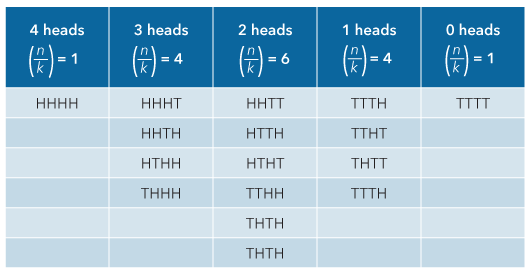Figure 5.14: Sample space of coin toss experiment Step 2: To complete the calculation and determine the probability of exactly 2 successes in 4 trials, you would multiply the combinations, 6, by the product of the probability of a success on a given trial, p, taken to the kth power, by the probability of a failure, q, taken to the n-k power. The formula, as you’ve seen above, is given as: Substituting, we get: The probability of getting exactly 2 heads during 4 tosses of a fair coin is .375. ### Discussion You may ask, “if the P of heads is .5, why isn’t the P of 2 heads in 4 tosses equal to .5?” To answer that, look at the sample space above. There, you can see that there are 16 possible outcomes given 4 trials of a binomial experiment. Six of those result in exactly 2 successes—in this case, heads coming up twice. The proportion of outcomes with 2 heads is equal to 6/16, or .375, which confirms the results of the formula-based calculation above. For practice, try this on a calculator that will figure binomials to confirm the values for and for P(k). (Some calculators may represent combinations as nCr, and some references may show it as C(n,r), but it’s all the same thing.) ### Probability of a Range of Outcomes of a Binomial Experiment You’ve now seen how to determine the probability associated with a particular outcome of a binomial trial. To compute a range of probabilities, you have to repeat and sum. Recall the addition rule, which tells us that the probability that one or another of two outcomes will occur is obtained by adding their respective probabilities¬—the sum of those individual probabilities equals the probability that one or the other will occur. This can be extended to multiple outcomes, so that the probability that one of several outcomes will occur is equal, again, to the sum of the individual probabilities. This can be summarized as Example 1 Referring again to the previous example of the probability of two heads in four tosses of a fair coin, we can also calculate the probability of any range of successful outcomes. For example, we could calculate the probability of 3 or fewer heads by using the addition rule, as follows: The probability of 3 or fewer heads, then, is Example 2 An easier way of figuring the above example would have been to simply subtract P(4) from 1. That would save a whole bunch of calculating! $\begin{array}{l}P(4)=P\begin{pmatrix}4\\4\end{pmatrix}(p)^k(q)^{n-k}=\frac{n!}{k!(n-k)!}\times(.5)^4(.5)^0=1(.0625)=.0625\\\\P(k\;\leq\;3)\;=\;1\;-\;P(4)\;=\;1\;-\;.0625\;=\;.9375\end{array}$ As a rule of thumb, any time the range of outcomes you want to figure the probability for is less than half of the possible values, add them up; if the range is more than half of the possible outcomes, figure the probabilities for the lesser number of outcomes and subtract from 1. 5.15 Which of the following is not needed to calculate the probability of k successes in n trials of a binomial trial? A The possible number of permutations B The binomial coefficient C The probability of success D The probability of failure 5.16 Calculate the probability of at least three successes in five trials where the probability of a success is 0.7. ## Mean, Variance and Standard Deviation of a Binomial The population parameters of a binomial distribution can be calculated using the formulas for any discrete probability distribution, which you learned in the first part of this lesson. They can be simplified for use with a binomial distribution. Example Refer to the previous example of the ways of getting two heads in four tosses of a fair coin. The mean of that distribution can be calculated as We can see by referring to the previous sample space table that two heads is indeed the most likely outcome. 5.17 What is the standard deviation of the distribution in the example just above? 5.18 What is the mean of the binomial distribution that consists of 10 trials where the probability of a success is 0.6? A 5 B 0.49 C 6 D 0.24 ### Question 5.19 5.19 How would you account for the fact that while the mean of the distribution in the example above is 2 heads, the probability associated with an outcome of 2 heads is 0.375? Click here to see the answer to Question 5.19. ## Distribution of Outcomes in Binomial Trials When the normal distribution was introduced you saw there how a bell-shaped curve can be used to graph many naturally occurring distributions of events. This same general pattern is also seen when graphing a series of outcomes of binomial trials. ### Examples: Distribution of Outcomes in Binomial Trials Example 1: Two Coins Suppose a friend asks you to play a game with a fair coin (one not at all biased towards landing heads-up or tails-up). The game is that you each start with a stack of 10 quarters. If it is your turn, you take one of your quarters, flip it, and call it in the air; if you correctly call the toss, you get the coin. Similarly, during your opponent’s turn, it is their coin that is used and their call, and there is an opportunity for you to win a quarter. Do you agree to play this game? If you’re okay with a game that is fair, you should take the game. On each coin toss, you have a 50:50 chance of prevailing. On each round of turns (each player tosses one of their own coins) you should, on average, lose as often as you win in the long run. So it’s just a sporting game, not a rip-off. Example 2: Four Coins Suppose you engaged in a game where for the price of a dollar, four quarters are tossed and you win four dollars if the coins are all heads or all tails. Is this a game where you are likely to win at least as much as you wagered? The simplest way to find out is to examine all possible outcomes.Figure 5.15: Sample space of a coin toss game.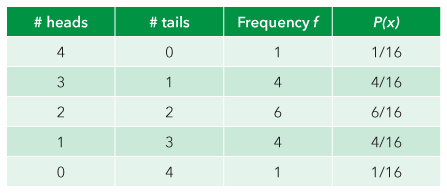Figure 5.16: Outcomes of a coin toss game​.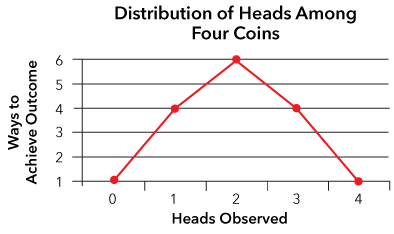Figure 5.17: Graph of outcomes of a coin toss game​. Notice that in graph format, the distribution of outcomes approximates a bell-shape. There are more ways to achieve two heads (or two tails, i.e., HHTT, HTHT, HTTH, THHT, THTH, TTHH) than there are ways to achieve any other combination of outcomes. There is only one outcome that yields four heads, and only one outcome that yields four tails. We will see that in a great many probability distributions, there are more paths toward the center of the distribution than there are to the extreme regions or tails of the distribution. To return to the original question, would you take this bet? You pay one dollar to get into the game, and you win four dollars if you get four heads or four tails. The probability of winning is 2/16ths or 1/8th. So on average, you’d win four dollars every time you played eight games, but you would have paid eight dollars to do so. In the long run, you’d pay twice as much as you won back. This would be a terrible game to put money on. ### The Multinomial Distribution The Binomial Distribution is limited to events or combinations of events where each event has only two possible outcomes. The Multinomial Distribution follows the same conceptual principles, but is necessary when individual events can take on more than two possible values. The roll of a single die is a very simple example. There are six possible outcomes. In such a simple case, the interpretation is fairly simple because each outcome is equally likely. In other situations, such as card playing, for example, there may be outcomes with varying probabilities as a function of preceding outcomes. In cases like this, the math involved in calculating probabilities can become very complex indeed. For simplicity, our examples will be restricted to throwing dice and doing so only as concepts, not computations. ### Examples: Distribution of Outcomes in a Multinomial Experiment Example 1: Rolling a Single Die Suppose the game is that a player pays a dollar to play, picks any two numbers, and if one of those two numbers comes up, that player gets$1.50 in return. Below is a chart of what the outcomes look like. Not very surprising or informative, but it does show you that the distribution is uniform across possible outcomes, with each outcome having the same probability. These distributions, or close approximations thereof, are relatively common. For instance, if you examined the number of children in each grade at a particular school, you’d find something close enough to be categorized as a uniform distribution.

But back to our game. Clearly (use the addition rule here), the odds of getting one of your two choices is 1/3, but the payback is only $1.50. So over the long haul, you could expect to get about 75 cents for every game you played, and have paid$1 for the privilege of playing. Another sucker’s bet.

Example 2: Rolling Two Dice

We’ve just seen that a single coin toss results in a uniform distribution, while multiple coin tosses yield a bell-shaped distribution. We’ve also seen that a single roll of a die yields a uniform distribution. What, then would you expect would happen if you rolled two dice?

Now there are a total of 36 possible outcomes. There are six possible outcomes for each die, and combining those yields 6 x 6 = 36 possible outcomes of the two dice together. Again, the sample space helps to show this. In this table, the first number in each pair is the first die and the second is the second die. The total of the two dice is then shown in the frequency table and the probabilities summarized.Figure 5.20: Frequency Table of Sums when Throwing Two Dice ​

Note: In the chart of the distribution below that the distribution is not exactly bell-shaped, but a lot closer than the distribution of throwing a single die.

Example 3: Rolling Three Dice

Recall that when the coin throw experiment was bell-shaped, we used four coins, not two. It would, then, be sensible to suppose that if we threw three dice, we might get something closer to a bell-shaped distribution. There are 216 outcomes with three dice, so they won’t all be listed, but you can see in the figure below what the outcomes look like for three dice, with scores ranging from 3-18.

Now, this looks a lot more bell-shaped! We will see on numerous occasions that more values produce a distribution shape that is more like a normal distribution.

For combined distributions (in this case, individual distributions for three dice), a sufficient number of distributions of any form (in this case, uniform distributions) always combine to be normal, and the more distributions and the more scores there are, the better the distribution.

5.20

The defining difference between a binomial distribution and a multinomial distribution is that in a multinomial distribution $\_\_\_\_\_\_\_\_\_\_\_$.

A

Each trial has two or fewer possible outcomes

B

Each trial has more than two possible outcomes

C

Each trial is independent of the other trials

D

Variables must be discrete values

5.21

A distribution of outcomes determined by drawing from a deck of cards to see if a King is drawn is an example of a $\_\_\_\_\_\_\_\_$ distribution.

## Distributions When Events Are Determined by Multiple Factors

We’ve used several examples to show that no matter the distribution of individual scores, scores determined by multiple events tend toward a bell-shaped distribution for a fairly straightforward reason. The only path to extreme scores is when all of the individual events separately head towards extreme scores, meaning that there are very few combinations of events that lead to extreme scores. But if some events go one way and others go the other, the outcome is heading towards a middle score, and there are many, many more paths to those middle scores; the more events enter into the combined score, the more paths there are to middle scores.

We’ll see more about this when the Law of Large Numbers and the Central Limit Theorem are introduced!

## Case Study: Identity Theft

According to a U.S. Department of Justice report released on September 27, 2015, an estimated 17.6 million persons, or about 7% of U.S. residents age 16 or older, were victims of at least one incident of identity theft in 2014. These results are similar to those seen for the previous two years.

“The most common type of identity theft was the unauthorized misuse or attempted misuse of an existing account—experienced by 16.4 million persons. Victims may have experienced multiple types of identity theft. An estimated 8.6 million victims experienced the fraudulent use of a credit card, 8.1 million experienced the unauthorized or attempted use of existing bank accounts (checking, savings or other) and 1.5 million victims experienced other types of existing account theft, such as misuse or attempted misuse of an existing telephone, online or insurance account.”

Source: 17.6 Million U.S. Residents Experienced Identity Theft In 2014. Bureau of Justice Statistics. Retrieved from http://bjs.ojp.usdoj.gov/index.cfm?ty=pbdetail&iid=5410.

Case Study Questions 5.01

Given the data above, what would be the probability of a person becoming a victim of any type of identity theft?

A

.07

B

.05

C

.03

D

.02

Case Study Question 5.02

Given the data above, what would be the probability of a person becoming a victim of credit card fraud? (Rounded to two decimals)

A

.07

B

.05

C

.03

D

.02

### Case Study Question 5.03

Case Study Question 5.03

Describe one way the data from the report could be used as the basis for a binomial experiment.

### Case Study Question 5.04

Case Study Question 5.04

Assign values for p and q and carry out the calculations for the outcome of the experiment described in your response to Question 5.03.

## Pre-Class Discussion Questions

### Class Discussion 5.01

Class Discussion 5.01

What is a random variable?

### Class Discussion 5.02

Class Discussion 5.02

Distinguish between discrete and continuous random variables.

### Class Discussion 5.03

Class Discussion 5.03

Distinguish between permutations and combinations.

### Class Discussion 5.04

Class Discussion 5.04

Describe and provide an example of a binomial distribution.

### Class Discussion 5.05

Class Discussion 5.05

Why is it that when a score is determined by multiple events, it often tends toward a bell-shaped distribution? Why not, say, a uniform distribution?This Content is Locked
Only a limited preview of this text is available. You'll need to sign up to Top Hat, and be a verified professor to have full access to view and teach with the content.

The mean of the distribution is two heads, indicating that is the most likely outcome out of the 16 possible outcomes. Out of those 16 possible outcomes, there are six ways of getting two heads, while there are 10 ways of getting an outcome other than two heads. We can determine the probability of either of those outcomes, either two heads or not two heads, by calculating the proportion of outcomes that meet either criterion. The probability of two heads, then, is equal to 6 / 16 = 0.375, and the probability of not two heads is equal to 10 / 16 = 0.625. Those two probabilities sum to one, confirming that those two sets of outcomes account for all possible outcomes of that probability experiment.

## Answers to Case Study Questions

### Answer to Case Study Question 5.03

If we were to randomly select 1000 people from a pool representing the general population, what would be the probability that at least 50 of those would have experienced some form of identity theft in the past year?

### Answer to Case Study Question 5.04

p = .07, n = 1000

P(k ≥ 50) = .996

## Answers to Pre-Class Discussion Questions

### Answer to Class Discussion 5.01

A random variable is a numerical value representing the outcome of a probability experiment. It is a random variable if the value it takes on is determined entirely by chance factors.

### Answer to Class Discussion 5.02

A continuous random variable, like height, has an uncountable number of possible outcomes, but it can be measured.

A discrete random variable, like the number of candies in a package, has outcomes that can be counted.

### Answer to Class Discussion 5.03

A permutation is a set of k items chosen from a set of n items without replacement and where order does matter. It is a specific arrangement of all or part of the n items.

A combination is a set of k items chosen from a set of n items without replacement and where the order does not matter.

### Answer to Class Discussion 5.04

The Binomial Distribution is a probability distribution summarizing outcomes of a binomial experiment, each trial of which has two and only two possible outcomes and must satisfy certain conditions. For example, the distribution of outcomes of tossing a coin constitutes a binomial distribution.